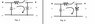# DC RC-Circuit Question.

• Engineering
DarkStalker
1. In the network given (Fig.1), the initial voltage on C1 is V1 and on C2 is V2 such that v1(0)=V1 and v2(0)=V2. At t=0 the switch K is closed.
(a) Find i(t) fo all time.
(b) Find v1(t) for t>0.
(c) Find v2(t) for t>0.
(d) From your results on (b) and (c), show that v1(∞)=v2(∞).

V=iR.
V=i/C∫i.dt

## The Attempt at a Solution

I'm lost on this one. I don't fully understand how to construct the equation for part (a). Is it:
i/C1∫i.dt-i/C2∫i.dt+iR=0
Or
i/C1∫i.dt+i/C2∫i.dt+iR=0

I'm having trouble understanding which one is the voltage drop and which one is the rise. Also, shouldn't the orientation of the capacitors also matter? For example, if we flip the capacitor C2 as shown in Fig. 2, how would the equations change?

#### Attachments

•Untitled.jpg
9.5 KB · Views: 390

Mentor
1. In the network given (Fig.1), the initial voltage on C1 is V1 and on C2 is V2 such that v1(0)=V1 and v2(0)=V2. At t=0 the switch K is closed.
(a) Find i(t) fo all time.
(b) Find v1(t) for t>0.
(c) Find v2(t) for t>0.
(d) From your results on (b) and (c), show that v1(∞)=v2(∞).

V=iR.
V=i/C∫i.dt

## The Attempt at a Solution

I'm lost on this one. I don't fully understand how to construct the equation for part (a). Is it:
i/C1∫i.dt-i/C2∫i.dt+iR=0
Or
i/C1∫i.dt+i/C2∫i.dt+iR=0

I'm having trouble understanding which one is the voltage drop and which one is the rise. Also, shouldn't the orientation of the capacitors also matter? For example, if we flip the capacitor C2 as shown in Fig. 2, how would the equations change?

Start by assigning variable names to the various potentials, with assumed orientation (polarity). So VC1, VR, VC2. Also choose an assumed direction for i in the loop (which you've done). Then you can write KVL in terms of those variables.

Now take each of those variables and expand them according to the given information. So, for example, for C1:
$$V_{C1} = V1 - \frac{1}{C1}\int_{I_o}^{I} i\;dt$$
Do the same for R and C2. Solve the equation.

The orientation of the capacitor symbol does not affect the circuit operation in any way. In general capacitance as a property is non-directional.

This is not to say that there aren't certain types of capacitors whose physical/chemical makeup require that voltage be oriented in a certain way on them (e.g. so-called electrolytic capacitors). Incorrect polarity will cause a chemical reaction that will degrade and eventually ruin them. So long as the voltage across these capacitors has the correct polarity, they provide the same capacitance no matter which direction the current flows (i.e. charging or discharging).